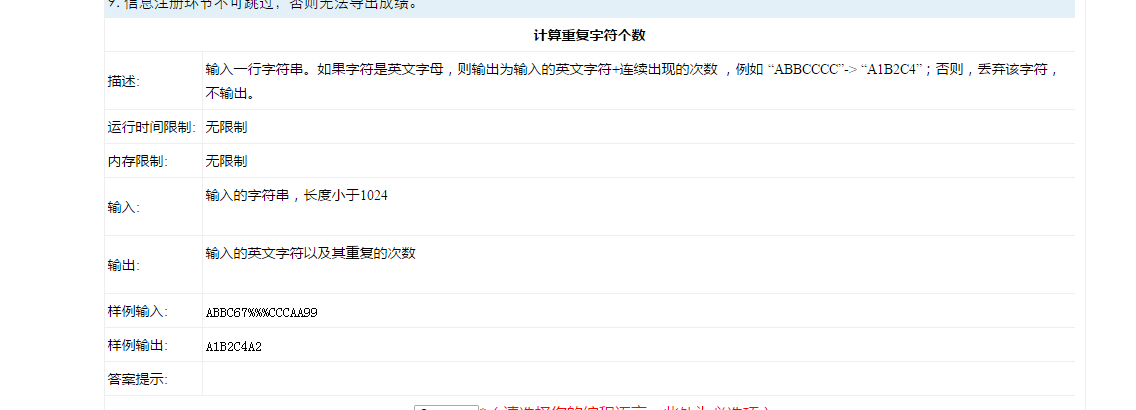#define _CRT_SECURE_NO_WARNINGS
#include
#include
#include
void main()
{
char str;
int num = {0};
scanf("%s", str);

for (int i = 0; i < strlen(str); i++)    //判断是否除了字母以外还有其他字符如果有则删去
{
while (str[i]<'A' || str[i]>'z' )
{
for (int j = i; j < strlen(str); j++)
{
str[j] = str[j + 1];
}
if (str[i] == '\0')
{
break;
}
}
}
int i = 0;
while (str[i]!='\0')
{
int count = 1;
int j = i;
while (str[j] == str[j + 1])
{
count++;
j++;
}
num[i] = count;
printf("%c", str[i]);
printf("%d", num[i]);
i += count;
}
//printf("%s", str);

system("pause");


6个回答

public class Test {
public static void main(String[] args) {
String s = "abbc65yyy&*ccc\$b1baa00";
StringBuffer sb = new StringBuffer();
char[] array = s.toCharArray();
char temp = '0';
int nowCharCount = 1;
for (int i = 0; i < array.length; i++) {
char c = array[i];
if (isEnglish(c)) {
//如果刚才出现了字符c
if (temp == c) {
sb.deleteCharAt(sb.length() - 1);
sb.append(nowCharCount + 1);
nowCharCount++;
continue;
}
nowCharCount = 1;
temp = c;
sb.append(c);
sb.append(nowCharCount);
} else {
//初始化
nowCharCount = 1;
temp = '0';
}
}

    System.out.println(sb.toString());
}

private static boolean isEnglish(char c) {
if ('a' <= c && c <= 'z') {
return true;
}
return false;
}


}4 年多之前 回复